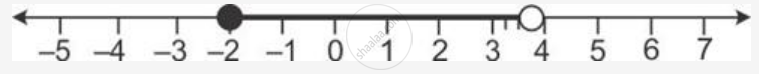Share

Solve the Following Inequation and Represent the Solution Set on the Number Line -3 < -1/2 - (2x)/3 ,= 5/6, X in R - ICSE Class 10 - Mathematics

ConceptRepresentation of Solution on the Number Line

Question

Solve the following inequation and represent the solution set on the number line

-3 < -1/2 - (2x)/3 ≤ 5/6, x in R

Solution

-3 < -1/2 - (2x)/3 <= 5/6

Multiply by 6 we get

=> -18 < -3 - 4x <= 5

=> -15 < - 4x <= 8

Dividing by -4 we get

=> (-15)/(-4)  > x >= 8/(-4)

=> -2 <=  x < 15/4

=> x  ∈  [-2,15/4]

The solution set can be represented on a number line as:Is there an error in this question or solution?

APPEARS IN

Solution Solve the Following Inequation and Represent the Solution Set on the Number Line -3 < -1/2 - (2x)/3 ,= 5/6, X in R Concept: Representation of Solution on the Number Line.
S